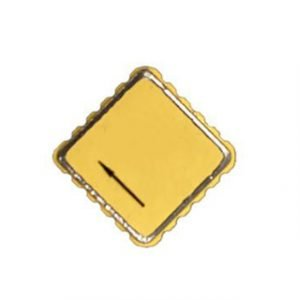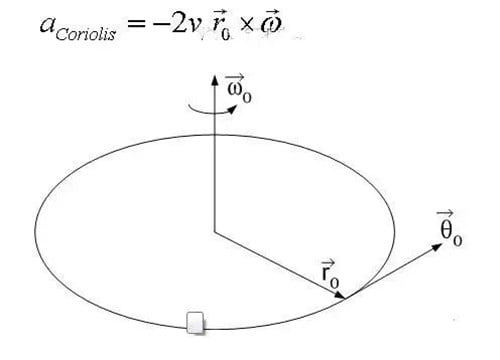Application

# How to Distinguish MEMS Accelerometer and MEMS Gyroscope Correctly?MEMS gyroscope measures angular velocity, and MEMS acceleration measures linear acceleration. The former is the principle of inertia, and the latter is the principle of force balance. The measured value of accelerometer is correct in a long time, but there is error in a short time due to the existence of signal noise. Gyroscopes are more accurate in a short time, and there will be errors due to drift in a long time. Therefore, both (mutual adjustment) are required to ensure correct heading. Now general inertial applications in attitude, such as IMU (Inertial Measurement Unit), are composed of three-axis gyroscope and three-axis accelerometer.

There are three kinds of with mature technology: piezoelectric, capacitive, and thermal. Piezoelectric MEMS accelerometer uses piezoelectric effect. There is a mass block supported by a rigid body inside the accelerometer. When there is movement, the mass block will generate pressure, the rigid body will generate strain, and the acceleration will be converted into electrical signal output.

There is also a mass block inside the capacitive MEMS accelerometer. From a single unit, it is a standard flat capacitor. The change of acceleration drives the moving mass block to change the distance between the two poles of the plate capacitor and the positive area. The acceleration is calculated by measuring the capacitance change.

There is no mass block inside the thermal MEMS accelerometer. There is a heating body in the center, a temperature sensor around, and a closed air chamber inside. Under the action of the heating body during operation, the gas forms a hot air mass inside. The specific gravity of the hot air mass is different from that of the surrounding cold air

The change of thermal field caused by the movement of hot air mass makes the sensor sense the acceleration value. Due to the existence of rigid body support inside the piezoelectric MEMS accelerometer, usually, the piezoelectric MEMS accelerometer can only sense the “dynamic” acceleration, but not the “static” acceleration, that is, the gravitational acceleration. The capacitive type and thermal type can sense both “dynamic” acceleration and “static” acceleration.

From the above analysis, we can see that when using capacitive and thermal accelerometers for orientation, the acceleration measured by the accelerometer includes the gravity component of the gravity acceleration on each axis and the acceleration component caused by dynamic motion. Therefore, I think we must remove the dynamic acceleration when using this type of accelerometer for orientation (it is more difficult). When detecting the motion of the chip, the gravitational acceleration must be removed.

MEMS gyroscopes use Coriolis force, the tangential force that a rotating object receives when it has radial motion. Assuming that the rotating object has a radial velocity Vr, the tangential Coriolis acceleration will be generated.From the above formula, we can see that the Coriolis acceleration is proportional to the angular velocity of the object rotation. If we install an accelerometer in the tangential direction to measure the Coriolis acceleration, then we can indirectly obtain the angular velocity of the object rotation.

Therefore, in the design of a MEMS gyroscope, the object is driven to move radially or vibrate continuously back and forth, and the corresponding Coriolis force is constantly changing back and forth in the horizontal direction, which may cause the object to vibrate slightly in the horizontal direction, and the phase is exactly 90 degrees away from the driving force. MEMS gyroscopes usually have movable capacitor plates in two directions. The radial capacitive plate plus the oscillating voltage forces the object to move radially (a bit like the self-test mode in the accelerometer), and the transverse capacitive plate measures the capacitance change due to the transverse Coriolis motion (just like the accelerometer measuring the acceleration). Since the Coriolis force is proportional to the angular velocity, the angular velocity can be calculated from the change of capacitance.